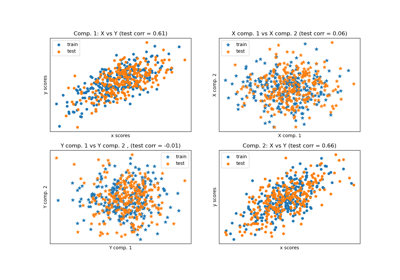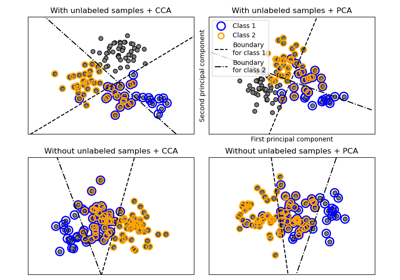# sklearn.cross_decomposition.CCA¶

class sklearn.cross_decomposition.CCA(n_components=2, *, scale=True, max_iter=500, tol=1e-06, copy=True)[source]

CCA Canonical Correlation Analysis.

CCA inherits from PLS with mode=”B” and deflation_mode=”canonical”.

Read more in the User Guide.

Parameters
n_componentsint, (default 2).

number of components to keep.

scaleboolean, (default True)

whether to scale the data?

max_iteran integer, (default 500)

the maximum number of iterations of the NIPALS inner loop

tolnon-negative real, default 1e-06.

the tolerance used in the iterative algorithm

copyboolean

Whether the deflation be done on a copy. Let the default value to True unless you don’t care about side effects

Attributes
x_weights_array, [p, n_components]

X block weights vectors.

y_weights_array, [q, n_components]

Y block weights vectors.

x_scores_array, [n_samples, n_components]

X scores.

y_scores_array, [n_samples, n_components]

Y scores.

x_rotations_array, [p, n_components]

X block to latents rotations.

y_rotations_array, [q, n_components]

Y block to latents rotations.

coef_array of shape (p, q)

The coefficients of the linear model: Y = X coef_ + Err

n_iter_array-like

Number of iterations of the NIPALS inner loop for each component.

Notes

For each component k, find the weights u, v that maximizes max corr(Xk u, Yk v), such that |u| = |v| = 1

Note that it maximizes only the correlations between the scores.

The residual matrix of X (Xk+1) block is obtained by the deflation on the current X score: x_score.

The residual matrix of Y (Yk+1) block is obtained by deflation on the current Y score.

References

Jacob A. Wegelin. A survey of Partial Least Squares (PLS) methods, with emphasis on the two-block case. Technical Report 371, Department of Statistics, University of Washington, Seattle, 2000.

In french but still a reference: Tenenhaus, M. (1998). La regression PLS: theorie et pratique. Paris: Editions Technic.

Examples

>>> from sklearn.cross_decomposition import CCA
>>> X = [[0., 0., 1.], [1.,0.,0.], [2.,2.,2.], [3.,5.,4.]]
>>> Y = [[0.1, -0.2], [0.9, 1.1], [6.2, 5.9], [11.9, 12.3]]
>>> cca = CCA(n_components=1)
>>> cca.fit(X, Y)
CCA(n_components=1)
>>> X_c, Y_c = cca.transform(X, Y)


Methods

 fit(self, X, Y) Fit model to data. fit_transform(self, X[, y]) Learn and apply the dimension reduction on the train data. get_params(self[, deep]) Get parameters for this estimator. inverse_transform(self, X) Transform data back to its original space. predict(self, X[, copy]) Apply the dimension reduction learned on the train data. score(self, X, y[, sample_weight]) Return the coefficient of determination R^2 of the prediction. set_params(self, \*\*params) Set the parameters of this estimator. transform(self, X[, Y, copy]) Apply the dimension reduction learned on the train data.
__init__(self, n_components=2, *, scale=True, max_iter=500, tol=1e-06, copy=True)[source]

Initialize self. See help(type(self)) for accurate signature.

fit(self, X, Y)[source]

Fit model to data.

Parameters
Xarray-like of shape (n_samples, n_features)

Training vectors, where n_samples is the number of samples and n_features is the number of predictors.

Yarray-like of shape (n_samples, n_targets)

Target vectors, where n_samples is the number of samples and n_targets is the number of response variables.

fit_transform(self, X, y=None)[source]

Learn and apply the dimension reduction on the train data.

Parameters
Xarray-like of shape (n_samples, n_features)

Training vectors, where n_samples is the number of samples and n_features is the number of predictors.

yarray-like of shape (n_samples, n_targets)

Target vectors, where n_samples is the number of samples and n_targets is the number of response variables.

Returns
x_scores if Y is not given, (x_scores, y_scores) otherwise.
get_params(self, deep=True)[source]

Get parameters for this estimator.

Parameters
deepbool, default=True

If True, will return the parameters for this estimator and contained subobjects that are estimators.

Returns
paramsmapping of string to any

Parameter names mapped to their values.

inverse_transform(self, X)[source]

Transform data back to its original space.

Parameters
Xarray-like of shape (n_samples, n_components)

New data, where n_samples is the number of samples and n_components is the number of pls components.

Returns
x_reconstructedarray-like of shape (n_samples, n_features)

Notes

This transformation will only be exact if n_components=n_features

predict(self, X, copy=True)[source]

Apply the dimension reduction learned on the train data.

Parameters
Xarray-like of shape (n_samples, n_features)

Training vectors, where n_samples is the number of samples and n_features is the number of predictors.

copyboolean, default True

Whether to copy X and Y, or perform in-place normalization.

Notes

This call requires the estimation of a p x q matrix, which may be an issue in high dimensional space.

score(self, X, y, sample_weight=None)[source]

Return the coefficient of determination R^2 of the prediction.

The coefficient R^2 is defined as (1 - u/v), where u is the residual sum of squares ((y_true - y_pred) ** 2).sum() and v is the total sum of squares ((y_true - y_true.mean()) ** 2).sum(). The best possible score is 1.0 and it can be negative (because the model can be arbitrarily worse). A constant model that always predicts the expected value of y, disregarding the input features, would get a R^2 score of 0.0.

Parameters
Xarray-like of shape (n_samples, n_features)

Test samples. For some estimators this may be a precomputed kernel matrix or a list of generic objects instead, shape = (n_samples, n_samples_fitted), where n_samples_fitted is the number of samples used in the fitting for the estimator.

yarray-like of shape (n_samples,) or (n_samples, n_outputs)

True values for X.

sample_weightarray-like of shape (n_samples,), default=None

Sample weights.

Returns
scorefloat

R^2 of self.predict(X) wrt. y.

Notes

The R2 score used when calling score on a regressor uses multioutput='uniform_average' from version 0.23 to keep consistent with default value of r2_score. This influences the score method of all the multioutput regressors (except for MultiOutputRegressor).

set_params(self, **params)[source]

Set the parameters of this estimator.

The method works on simple estimators as well as on nested objects (such as pipelines). The latter have parameters of the form <component>__<parameter> so that it’s possible to update each component of a nested object.

Parameters
**paramsdict

Estimator parameters.

Returns
selfobject

Estimator instance.

transform(self, X, Y=None, copy=True)[source]

Apply the dimension reduction learned on the train data.

Parameters
Xarray-like of shape (n_samples, n_features)

Training vectors, where n_samples is the number of samples and n_features is the number of predictors.

Yarray-like of shape (n_samples, n_targets)

Target vectors, where n_samples is the number of samples and n_targets is the number of response variables.

copyboolean, default True

Whether to copy X and Y, or perform in-place normalization.

Returns
x_scores if Y is not given, (x_scores, y_scores) otherwise.

## Examples using sklearn.cross_decomposition.CCA¶